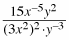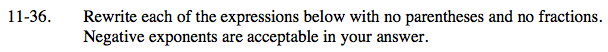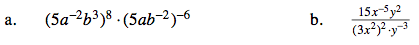Home > AC > Chapter 11 > Lesson 11.1.3 > Problem11-36

11-36.
1. Rewrite each of the expressions below with no parentheses and no fractions. Negative exponents are acceptable in your answer. Homework Help ✎

1. (5a−2b 3)8 · (5ab−2)−6

2.Distribute the exponents.

(58a−16b24) · (5−6a−6b12)

How many factors of 5 are multiplied together? How many of a? How many of b?

25a−22b36

Simplify the denominator first.

Write 3x2 twice and find how many factors of 3 are multiplied together and how many of x are multiplied together.

Now think about making ones and where any left over factors would remain.